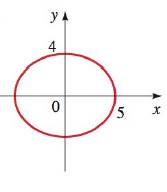Chapter 12.2, Problem 29E### Algebra and Trigonometry (MindTap ...

4th Edition
James Stewart + 2 others
ISBN: 9781305071742

#### Solutions

Chapter
Section### Algebra and Trigonometry (MindTap ...

4th Edition
James Stewart + 2 others
ISBN: 9781305071742
Textbook Problem

# 2 9 - 3 4 . Finding the equation of an ellipse Find the equation of an ellipse whose graph is shown below.To determine

To find:

The equation of an ellipse whose graph is given.

Explanation

Given:

Figure (1)

That is the value a=5 and the value of b=4.

Approach:

For an ellipse with the horizontal major axis

x2a2+y2b2=1,a>0,b>0...(1)

The vertices are (±a, 0)

### Still sussing out bartleby?

Check out a sample textbook solution.

See a sample solution

#### The Solution to Your Study Problems

Bartleby provides explanations to thousands of textbook problems written by our experts, many with advanced degrees!

Get Started